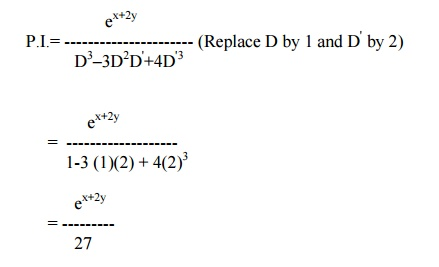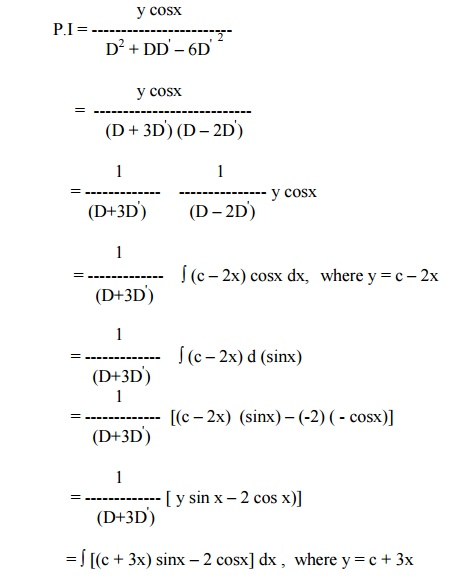Home | | Transforms and Partial Differential Equations | Partial Differential Equations of Higher Order With Constant Coefficients

# Partial Differential Equations of Higher Order With Constant Coefficients

Homogeneous Linear Equations with constant Coefficients. A homogeneous linear partial differential equation of the nth order is of the form

PARTIAL DIFFERENTIAL EQUATIONS OF HIGHER ORDER WITH CONSTANT COEFFICIENTS.

Homogeneous Linear Equations with constant Coefficients.

A homogeneous linear partial differential equation of the nth order is of the formhomogeneous because all its terms contain derivatives of the same order.

Equation (1) can be expressed asAs in the case of ordinary linear equations with constant coefficients the complete solution of (1) consists of two parts, namely, the complementary function and the particular integral.

The complementary function is the complete solution of f (D,D') z = 0-------(3), which must contain n arbitrary functions as the degree of the polynomial f(D,D'). The particular integral is the particular solution of equation (2).

Finding the complementary function

Let us now consider the equation f(D,D') z = F (x,y)

The auxiliary equation of (3) is obtained by replacing D by m and D' by 1.Solving equation (4) for â€žmâ€ź, we get â€žnâ€ź roots. Depending upon the nature of the roots, the Complementary function is written as given below:Finding the particular IntegralExpand [f (D,D')]-1 in ascending powers of D or D' and operate on xm yn term by term.

Case (iv) : When F(x,y) is any function of x and y.into partial fractions considering f (D,D') as a function of D alone.

Then operate each partial fraction on F(x,y)  in such a way thatwhere c is replaced by y+mx after integration

Example 26

Solve(D3 â€“3D2D' + 4D'3) z = ex+2y

The auxillary equation is m=m3 â€“3m2 + 4 = 0

The roots are m = -1,2,2

Therefore the C.F is f1(y-x) + f2 (y+ 2x) + xf3 (y+2x).Hence, the solution is z  = C.F. + P.IExample 27

Solve (D2 â€“4DD' +4 D' 2) z  = cos (x â€“2y)

The auxiliary equation is m2 â€“4m + 4 = 0

Solving, we get m = 2,2.

Therefore the        C.F is f1(y+2x) + xf2(y+2x).Example 28

Solve (D2 â€“2DD') z = x3y + e5x

The auxiliary equation is m2 â€“2m = 0.

Solving, we get     m = 0,2.

Hence the    C.F    is       f1 (y) + f2 (y+2x).Example 29The auxiliary equation is m2 + m â€“6 = 0.

Therefore,   m = â€“3, 2.

Hence the C.F is   f1(y-3x) + f2(y + 2x).=   Ă˛(c + 3x) d(â€“cosx) â€“2Ă˛cosx  dx

= (c + 3x) (â€“cosx) â€“(3) ( - sinx) â€“2 sinx

=   â€“y cosx + sinx

Hence the complete solution is

z = f1(y â€“3x) + f2(y + 2x) â€“y cosx + sinx

Example 30

Solve r â€“4s + 4t = e 2x +yi.e, (D2 â€“4DD' + 4D' 2  ) z = e2x + y

The auxiliary equation is m2 â€“4m + 4 = 0.

Therefore, m = 2,2

Hence the C.F is f1(y + 2x) + x f2(y + 2x).Since D2 â€“4DD'+4D'2 = 0 for D = 2  and D' = 1, we have to apply the general rule.Study Material, Lecturing Notes, Assignment, Reference, Wiki description explanation, brief detail
Mathematics (maths) : Partial Differential Equations : Partial Differential Equations of Higher Order With Constant Coefficients |